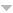431 sonucu aktar:
Yazar Başlık Tür [ Yıl]
Süzgeçler: Filter:   [Clear All Filters]
2020
Journal of the Operational Research Society, vol. 71, pp. 2042-2052, 2020.
Computers & operations research, vol. 123, pp. 104996, 2020.
2019
Computers & Operations Research, vol. 111, pp. 214-229, 2019.
Annals of Operations Research, vol. 279, pp. 1–42, 2019.
Turkish Journal of Electrical Engineering & Computer Sciences, vol. 27, pp. 832–846, 2019.
Physics in Medicine {&} Biology, vol. 64, pp. 205024, oct, 2019.
Şeker, O., T. Ekim, and Z. C. Taşkın, Networks, vol. 73, pp. 145-169, 2019.
European Journal of Operational Research, vol. 272, pp. 372-388, 2019.
Deniz, Z., and T. Ekim, Discrete Applied Mathematics, vol. 261, pp. 136-147, 2019.
Gürel, Y., and R. Güllü, International Journal of Production Economics, vol. 209, pp. 112-120, 2019.
IEEE Transactions on Intelligent Transportation Systems, vol. 20, pp. 4635-4642, 2019.
Edali, M., and G. Yücel, Simulation Modelling Practice and Theory, vol. 92, pp. 62-81, 2019.
European Journal of Operational Research, vol. 278, pp. 105-119, 2019.
Döyen, A., and N. Aras, Networks and Spatial Economics, vol. 19, pp. 1031–1068, 2019.
Discrete Applied Mathematics, vol. 261, pp. 2-21, 2019.
IISE Transactions, vol. 51, pp. 1061-1074, 2019.
INTERNATIONAL JOURNAL OF INDUSTRIAL ENGINEERING-THEORY APPLICATIONS AND PRACTICE, vol. 26, pp. 0–0, 2019.
European Journal of Operational Research, vol. 277, pp. 62-83, 2019.
Future Generation Computer Systems, vol. 101, pp. 959-974, 2019.
Ekim, T., J. Gimbel, and O. Şeker, Discrete Optimization, vol. 34, pp. 100548, 2019.
Computers & Operations Research, vol. 110, pp. 1-17, 2019.
2018
Discrete Mathematics & Theoretical Computer Science, vol. 20, 2018.
Edali, M., and G. Yücel, Journal of Artificial Societies and Social Simulation, vol. 21, pp. 1, 2018.
Pattern Recognition, vol. 73, pp. 202 - 215, 2018.
Simulation and Modeling Methodologies, Technologies and Applications, Cham, Springer International Publishing, 2018.
Küçükaydın, H., and N. Aras, Sigma, vol. 9, pp. 371–382, 2018.
Başoğlu, İ., W. Hörmann, and H. Sak, Annals of Operations Research, vol. 260, pp. 113–128, 2018.
Discrete Mathematics, vol. 341, pp. 2859-2871, 2018.
Discrete Mathematics and Theoretical Computer Science, vol. 20, pp. 1b–1b, 2018.
Metin, E., and G. Kumbaroğlu, International Journal of Energy Economics and Policy, vol. 8, pp. 141–149, 2018.